RSWebinar: Designing Cost-Effective Ground Improvement Solutions with Settle3 | June 7th, 2023 Register Now

# Geometry Overview

In Cpillar, the pillar geometry can be modelled as either a:

• Rectangular Pillar
• Polygonal Pillar

## Rectangular Pillar

### Pillar Dimensions

A Rectangular pillar is defined by its Length, Width, and Height, or x, y, and z values. These are the first 3 items entered in the Geometry tab of the Input Data dialog for Rigid, Elastic, or Voussoir analysis methods.

NOTE: Depending on the relative magnitudes of x, y, and z, the term "pillar", "beam", or "plate" may be most applicable, as described at the end of this section. For convenience, the term "pillar" is used most often throughout the Help.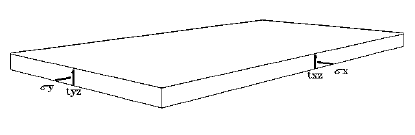Plate with dimensions x = 10, y = 20, z = 1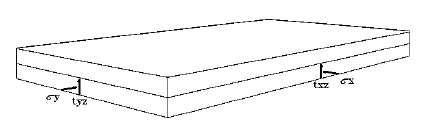Plate with dimensions x = 10, y = 20, z = 1; overburden thickness = 1Plate with dimensions x = 10, y = 20, z = 1; overburden thickness = 1, water height = 2.5

### Face Dip

For a Voussoir Analysis, the user can specify a Face Dip angle (degrees from horizontal) for the excavation face (>=0 and < 90). Specifying an angle > 0 has the effect of lowering the effective gravitational driving force by a factor of cos(dip angle). Other than this, the analysis procedure is the same as for a zero dip angle. See the Voussoir Analysis topic for more information.

## Polygonal Pillar

### Pillar Dimensions

A Polygonal pillar is defined by a at least three unique vertices (x, y), and a Height (z) value. The polygon geometry can be viewed and defined in the Stope Section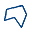view.

To define the vertices of the Polygonal Pillar in the Stope Section view, you can:

• Select Add Stope Section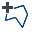option from the Boundaries menu, or right-click menu.
• Select Edit Coordinates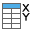option from the Edit sub-menu of the Boundaries menu, or right-click menu.

See the Stope Section, and Stope Section View topics for more information.

### Abutment Orientation

Non-vertical abutments can also be specified by changing the Abutment Trend and Abutment Plunge under the Geometry tab in Input Data dialog.

Polygon Pillar option and non-vertical abutments are only available in Rigid Analysis since in a Rigid Analysis only consider "plug" failure. Pillar Dimensions will be reset to rectangular entries, and Abutment Orientation will be reset to a Trend of 0 degrees, and Plunge of 90 degrees.

## Pillars, Beams, and Plates

Depending on the relative magnitudes of x, y, and z, the term pillar, beam, or plate will most correctly describe the geometric model:

• A pillar refers to a model with a low span/depth ratio, the span being the shorter of the x and y dimensions.
• A beam refers to a model with one of the lateral dimensions substantially longer than the other, and a fairly high span/depth ratio (e.g. > 3). Note that the beam span is the short lateral dimension.
• A plate refers to a model where both lateral dimensions are of similar magnitude, and a fairly high span/depth ratio (e.g. > 3).

To model the roof of a long excavation (a beam), make one lateral dimension much longer than the other (e.g. x = 10, y = 100). A factor of 10 should suffice, although the user can specify any realistic dimensions (e.g. x = 10000, y = 10).

The "long" and "short" lateral dimensions can be either the x or the y dimension. Make sure the corresponding lateral stresses are consistent with the chosen x and y directions.